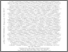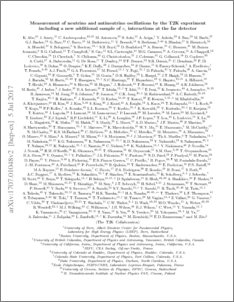# Measurement of neutrino and antineutrino oscillations by the T2K experiment including a new additional sample of $ν_e$ interactions at the far detector

Abe, K. and Amey, J. and Andreopoulos, C. and Antonova, M. and Aoki, S. and Ariga, A. and Ashida, Y. and Ban, S. and Barbi, M. and Barker, G. J. and Barr, G. and Barry, C. and Batkiewicz, M. and Berardi, V. and Berkman, S. and Bhadra, S. and Bienstock, S. and Blondel, A. and Bolognesi, S. and Bordoni, S. and Brailsford, D. and Bravar, A. and Bronner, C. and Avanzini, M. Buizza and Calland, R. G. and Campbell, T. and Cao, S. and Catanesi, M. G. and Cervera, A. and Chappell, A. and Checchia, C. and Cherdack, D. and Chikuma, N. and Christodoulou, G. and Coleman, J. and Collazuol, G. and Coplowe, D. and Cudd, A. and Dabrowska, A. and Rosa, G. De and Dealtry, T. and Finch, A. J. and Knox, A. and Kormos, L. L. and Lamont, I. and Lawe, M. and Nowak, J. and O'Keeffe, H. M. and Ratoff, P. N. and Shaw, D. (2017) Measurement of neutrino and antineutrino oscillations by the T2K experiment including a new additional sample of $ν_e$ interactions at the far detector. Physical Review D, 96 (9). ISSN 1550-7998Preview
PDF (1707.01048v2)
1707.01048v2.pdf - Accepted Version
The T2K experiment reports an updated analysis of neutrino and antineutrino oscillations in appearance and disappearance channels. A sample of electron neutrino candidates at Super-Kamiokande in which a pion decay has been tagged is added to the four single-ring samples used in previous T2K oscillation analyses. Through combined analyses of these five samples, simultaneous measurements of four oscillation parameters, $|\Delta m^2_{32}|$, $\sin^2(\theta_{23})$, $\sin^2(\theta_{13})$, and $\delta_{CP}$ and of the mass ordering are made. A set of studies of simulated data indicates that the sensitivity to the oscillation parameters is not limited by neutrino interaction model uncertainty. Multiple oscillation analyses are performed, and frequentist and Bayesian intervals are presented for combinations of the oscillation parameters with and without the inclusion of reactor constraints on $\sin^2(\theta_{13})$. When combined with reactor measurements, the hypothesis of CP conservation ($\delta_{CP}$$=0$ or $\pi$) is excluded at 90% confidence level. The 90% confidence region for $\delta_{CP}$ is [-2.95,-0.44] ([-1.47, -1.27]) for normal (inverted) ordering. The central values and 68\% confidence intervals for the other oscillation parameters for normal (inverted) ordering are $\Delta m^{2}_{32}=2.54\pm0.08$ ($2.51\pm0.08$) $\times 10^{-3}$ eV$^2 / c^4$ and $\sin^2(\theta_{23}) = 0.55^{+0.05}_{-0.09}$ ($0.55^{+0.05}_{-0.08}$), compatible with maximal mixing. In the Bayesian analysis, the data weakly prefer normal ordering (Bayes factor 3.7) and the upper octant for $\sin^2(\theta_{23})$ (Bayes factor 2.4).View Item